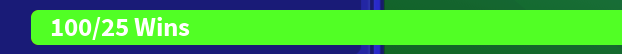# Progress bar problem

I got a progress bar and everytime the player gets past the the 25 Wins the green bar gets like thisIs there a way to cap the green bar?

Thats the local script:

``````local player = game.Players.LocalPlayer
local Wins = player.mFolder.mWins
while true do wait()
script.Parent.Progress.Bar:TweenSize(UDim2.new(0,(Wins.Value/ 25 * 195),1,0), "Out","Linear")
script.Parent.Progress.Pro3.Text = Wins.Value.."/"..game.Players.LocalPlayer.PlayerGui.Main.Main:WaitForChild("MainFrame").Items:WaitForChild("1").Top.Cost.Text

end``````

You would use `math.clamp` to cap a Value under a certain amount.

`math.max` if you want to cap it at a low value

``````local n  = -1
local min = 0

print(math.max(m, min)) -- 0 is higher than -1, automatically sets to 0
``````

`math.min` if you want to cap it at a High Value

``````local n  = 100
local max = 99

print(math.min(n, max)) -- 99 is lower than 100, automatically sets to 99
``````

`math.clamp` would sort of be both. (sort of because `max` and `min` are not really meant for that purpose, but work)

``````print(math.clamp(n, min, max))
``````

You should Also use `.Changed` Instead of a `while` loop.

4 Likes
``````local player = game.Players.LocalPlayer
local Wins = player.mFolder.mWins
while true do wait()
script.Parent.Progress.Bar:TweenSize(UDim2.new(0,math.max(1, Wins.Value/ 25)* 195,1,0), "Out","Linear")
script.Parent.Progress.Pro3.Text = Wins.Value.."/"..game.Players.LocalPlayer.PlayerGui.Main.Main:WaitForChild("MainFrame").Items:WaitForChild("1").Top.Cost.Text

end``````

You are mixing scale and offset in your `UDim2` constructor i.e.

``````UDim2.new(0,(Wins.Value/ 25 * 195),1,0); -- scale x = 0, offset x = (Wins.Value/ 25 * 195), scale y = 1, offset y = 0.
``````

Use `UDim.fromScale()`, or `UDim2.fromOffset()` if you require one size/pos regime. It looks like you are using offset but mixed the two up by assigning them incorrectly.

To create the correct length of the bar (using offset) just multiply the length of the bar by the percentage of Wins to MaxWins, i.e.

``````local player = game.Players.LocalPlayer;
local Wins = player.mFolder.mWins;
local MaxWins = 100;
local ySize = 1;

while true do wait()
local progress = Wins.Value / MaxWins;
script.Parent.Progress.Bar:TweenSize(UDim2.fromOffset(progress,ySize), "Out","Linear", 1, false, nil); -- the last three parameters are being supplied as default so I added them to see what you are asking of the function
-- ... rest of code

end
``````

I cant figure it out how to use math.clamp in my script, could you maybe help with that